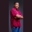Related Tags

int32
float
c#
type conversion
communitycreator

# What is Convert.ToInt32() in C#?Theodore Kelechukwu Onyejiaku

### Overview

Convert.ToInt32() converts a floating-point value (float, double) to a 32-bit integer value. We pass a floating-point value to this function, and it will output an Int32 type value.

### Syntax

Convert.ToInt32(floatValue).
Syntax for converting a float value to Int32 value

### Parameters

This method only takes a single parameter:

• floatValue: This is the floating-point value that we want to convert to a 32-bit integer.

### Return value

It returns an Int32 value corresponding to the floatValue. For example, if floatValue is 21.34, it will return 21.

### Code example

Let's look at the code below:

using System;
class HelloWorld
{
static void Main()
{
// create some floating point values
float floatVal = 3.0001F;
double doubleVal = 53.223;

// convert to Int32 and print results
Console.WriteLine("Float value: {0}, Int32 value: {1}", floatVal, Convert.ToInt32(floatVal));
Console.WriteLine("Double value: {0}, Int32 value: {1}", doubleVal, Convert.ToInt32(doubleVal));
}
}
Convert a float value to Int32 in C#

### Explanation

Let's look at the explanation of the code above:

• Lines 7 and 8: We create some variables with floating-point values.
• Lines 11 and 12: We use the Convert.ToInt32() method to convert each of the floating-point values to 32-bit integer values and print the results to the console.

RELATED TAGS

int32
float
c#
type conversion
communitycreator

CONTRIBUTORTheodore Kelechukwu Onyejiaku
RELATED COURSES

View all Courses

Keep Exploring

Learn in-demand tech skills in half the time